Flash - Distillation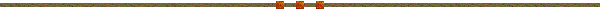This process occur when the product enter into a vessel.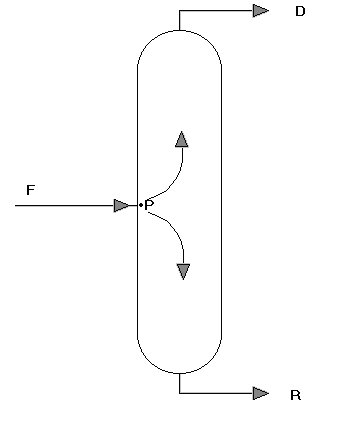The F mixture is in equilibrium, then it in the P point separate itself.

The vapor temperature can be considerate equal to the condensation temperature and the liquid temperature as the boil temperature.

When the P temperature is great than boil temperature then, all of F mixture is vapor, and the percent of vapor will be 100%

When the P temperature is less than condensation temperature, then all of F mixture is liquid, and the percent of vapor be 0%

but, if the P temperature is between the boil temperature and the condensation temperature, then part of F mixture is vapor and other par is liquid.

The percent of vapor can be calculated for the following equations :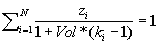where

zi is the molar fraction of each component of the F mixture.

ki is the equilibrium constant for the temperature in the P point that it can be considerate equal to the temperature of the F mixture.

Vol is the percent of vapor.

Then the percent of liquid must (1 - Vol ).

Vapor flow and  liquid flow will be :

D = F*Vol

R=F*(1-Vol)

Where :

F is the molar flow of the F mixture.

The products in D will be calculate with the following equation :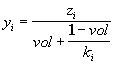where

yi is the molar fraction of each component of the D product.

zi is the molar fraction of each component of the F mixture.

ki is the equilibrium constant for the temperature in the P point that it can be considerate equal to the temperature of the F mixture.

Vol is the percent of vapor.

The products in R will be calculate with the following equation :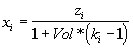xi is the molar fraction of each component of the R product.

zi is the molar fraction of each component of the F mixture.

ki is the equilibrium constant for the temperature in the P point that it can be considerate equal to the temperature of the F mixture.

Vol is the percent of vapor.ONLINE Program Flash Distillation it is more security and easy to use, it has 800 compounds.